## Specify Input Ranges on Simulink and Stateflow Elements

When you specify input range constraints on Simulink® and Stateflow® elements, Simulink Design Verifier™ considers these constraints during analysis.

### Specify Input Ranges for Inport Blocks

After you specify the output minimum and maximum values on Inport blocks, Simulink Design Verifier analysis uses the minimum and maximum values as constraints.

The following example model restricts the signals from two Inport blocks:

• Input1 block: Minimum: 1, Maximum: 5

• Input2 block: Minimum: -1, Maximum: 1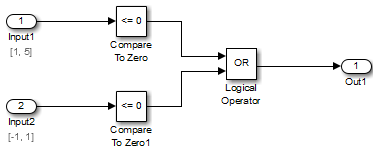When you use Simulink Design Verifier, to analyze this model, the analysis produces these results:

• The output from Input1 is never less than 0, therefore the first input to the Logical Operator block is never `false`. The objective that the first input to the Logical Operator equals `false` is unsatisfiable.

• The Logical Operator block cannot achieve 100% modified condition/decision coverage (MCDC) coverage because the condition where the first input is `false` never occurs.

The detailed analysis report shows the values you use as constraints for Input1 and Input2.

Note

Simulink Design Verifier considers the full range of possible values (and any minimum and maximum constraints) for root-level inports only.

### Specify Input Ranges for `Simulink.Signal` Objects

Using the Model Explorer, in the model workspace, you can specify minimum and maximum values on `Simulink.Signal` objects associated with input signals.

The following example model uses the `Simulink.Signal` objects associated with the input signals `a` and `b` to restrict the signal values:

• Signal `a`: Minimum: 1, Maximum: 5

• Signal `b`: Minimum: -1, Maximum: 1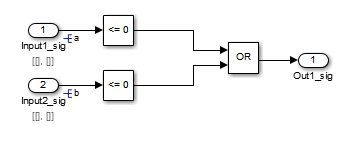When you analyze this model, the results are the same as if you specified the minimum and maximum values on the input ports.

#### Specifying Signal Ranges on Inport Blocks and Signals

If you specify ranges on the Inport blocks and on the signals, the analysis considers the smallest range for the values. For example, if you specify a range of `4..12` on an input port and a range of `1..8` on the signal from the input port, the analysis considers the range `4..8`.

### Specify Input Ranges for Stateflow Data Objects

Using the Model Explorer, you can specify ranges on data objects that are directly connected to the root-level input ports for a Stateflow chart.

In the following example model, the Stateflow chart named Chart has a data object, `x`, whose range you specified as 0 < `x` < 10. In this chart, `x` must be greater than 15 to trigger the transition from `low` to `high`.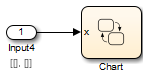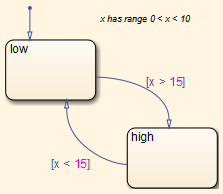The value of `x` ranges from 0 through 10, therefore the transition condition `[x > 15]` is never `true`. The transition from `low` to `high` never occurs. Because the `high` state is never entered, the transition condition `[x < 15]` is never tested, and the transition from `high` to `low` never occurs. The chart is always in the `low` state.

When you analyze this model, these objectives are proven unsatisfiable:

• The `high` state is never entered.

• The transition condition `[x > 15]` is always `false`, never `true`.

• The condition `[x < 15]` is never tested, so it is never `true` or `false`.

The analysis report indicates the values that you use as constraints for `x`: [0, 10].

### Specify Input Ranges for Subsystems

The Simulink Design Verifier software considers specified input minimum and maximum values as constraints only at the top level of a model. You can specify minimum and maximum values on Input ports on subsystems, but when you analyze the top-level model, the software ignores those values.

When you perform the subsystem analysis, the software considers specified minimum and maximum values on the input ports of the subsystem.

For example, consider the following model and its subsystem.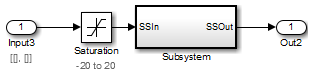In Subsystem, the specified minimum and maximum values for input port SSIn are -10 and 10, respectively. The lower and upper limits for the Saturation block are -15 and 15, respectively.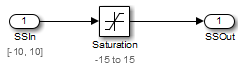If you right-click Subsystem in the top-level model and select Design Verifier > Generate Tests for Subsystem, the analysis considers the specified minimum and maximum values as constraints on the SSIn port.

Constraints: Design Min Max Constraints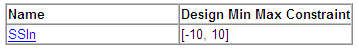The analysis identifies two unsatisfiable objectives:

• input > lower limit F: The input is always greater than the lower limit on the Saturation block (-15).

• input >= upper limit T: The input is never greater than or equal to the upper limit on the Saturation block (15).

If you analyze the model that contains Subsystem, the analysis does not consider the values specified on the input port SSIn in the subsystem. The analysis considers only the root-level input ports at the respective level of the hierarchy for analysis.

### Specify Input Ranges for Global Data Stores

A data store is a repository to which you can write data and from which you can read data, without having to connect an input or output signal directly to the data store. You create a data store by using a Data Store Memory block or a `Simulink.Signal` object. You can specify minimum and maximum values for any data store.

During subsystem analysis, Simulink Design Verifier creates an input port to mimic the execution context for a global data store. For more information, see Extract Subsystems for Analysis. If the data store has specified minimum and maximum values, those values are assigned as minimum and maximum values on the new input port. Simulink Design Verifier analysis considers the input minimum and maximum values as subsystem-level analysis constraints.

In the following example model, data store `A` has a minimum value of 0 and a maximum value of 10.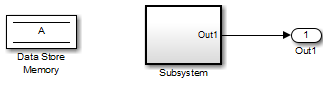The atomic subsystem reads values from the data store and checks to see if the input is less than `0`. The Compare To Zero block outputs 1 if the input is less than 0, and outputs 0 if the input is greater than or equal to 0. The Test Objective block checks to see if the output is ever 1.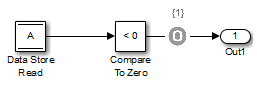In the top-level model, if you right-click Subsystem and select Design Verifier > Generate Tests for Subsystem, the analysis considers the constraints for data store `A` to be [0, 10].

The analysis does not satisfy the objective specified in the Test Objective block. The input is always greater than or equal to 0, therefore the output from the Compare To Zero block is always 0.

### Specify Input Ranges for Bus Elements

When you define a bus, you can specify minimum and maximum values for the elements in the bus. Simulink Design Verifier considers these minimum and maximum values when analyzing subsystems and models that use the bus as an input signal.

Consider a subsystem that inputs a bus of three fields, each with a defined minimum and maximum. To view this subsystem, at the command line, enter:

```open_system(fullfile(docroot,'toolbox','sldv','examples',... 'sldvBusMinMaxExample'));```Bus ElementBus Element MinimumBus Element Maximum
vehicleSpeed0125
throttle0100
engineSpeed07600

The subsystem has test objectives that confirm that each element does not exceed a constant. The `vehicleSpeed` signal is limited to a maximum value lower than the test objective.Set the current folder to a writable folder. In the top-level mode, right-click Subsystem and select Design Verifier > Generate Tests for Subsystem. The Condition Objective for testing ```vehicleSpeed > 135``` is not satisfiable due to the maximum specification on the `vehicleSpeed` element.